# Image Filtering

Back to index

## Introduction

Image filtering allows you to apply various effects on photos. The type of image filtering described here uses a 2D filter similar to the one included in Paint Shop Pro as User Defined Filter and in Photoshop as Custom Filter.

## Convolution

The trick of image filtering is that you have a 2D filter matrix, and the 2D image. Then, for every pixel of the image, take the sum of products. Each product is the color value of the current pixel or a neighbor of it, with the corresponding value of the filter matrix. The center of the filter matrix has to be multiplied with the current pixel, the other elements of the filter matrix with corresponding neighbor pixels.

This operation where you take the sum of products of elements from two 2D functions, where you let one of the two functions move over every element of the other function, is called Convolution or Correlation. The difference between Convolution and Correlation is that for Convolution you have to mirror the filter matrix, but usually it's symmetrical anyway so there's no difference.

The filters with convolution are relatively simple. More complex filters, that can use more fancy functions, exist as well, and can do much more complex things (for example the Colored Pencil filter in Photoshop), but such filters aren't discussed here.

The 2D convolution operation requires a 4-double loop, so it isn't extremely fast, unless you use small filters. Here we'll usually be using 3x3 or 5x5 filters.

There are a few rules about the filter:
• Its size has to be uneven, so that it has a center, for example 3x3, 5x5 and 7x7 are ok.
• It doesn't have to, but the sum of all elements of the filter should be 1 if you want the resulting image to have the same brightness as the original.
• If the sum of the elements is larger than 1, the result will be a brighter image, and if it's smaller than 1, a darker image. If the sum is 0, the resulting image isn't necessarily completely black, but it'll be very dark.
The image has finite dimensions, and if you're for example calculating a pixel on the left side, there are no more pixels to the left of it while these are required for the convolution. You can either use value 0 here, or wrap around to the other side of the image. In this tutorial, the wrapping around is chosen because it can easily be done with a modulo division.

The resulting pixel values after applying the filter can be negative or larger than 255, if that happens you can truncate them so that values smaller than 0 are made 0 and values larger than 255 are set to 255. For negative values, you can also take the absolute value instead.

In the Fourier Domain or Frequency Domain, the convolution operation becomes a multiplication instead, which is faster. In the Fourier Domain, much more powerful and bigger filters can be applied faster, especially if you use the Fast Fourier Transform. More about this is in the Fourier Transform article. In this article, we'll look at a few very typical small filters, such as blur, edge detection and emboss.

Image filters aren't feasible for real time applications and games yet, but they're useful in image processing.

Digital audio and electronic filters work with convolution as well, but in 1D.

Here's the code that'll be used to try out different filters. Apart from using a filter matrix, it also has a multiplier factor and a bias. After applying the filter, the factor will be multiplied with the result, and the bias added to it. So if you have a filter with an element 0.25 in it, but the factor is set to 2, all elements of the filter are in theory multiplied by two so that element 0.25 is actually 0.5. The bias can be used if you want to make the resulting image brighter.

The result of one pixel is stored in floats red, green and blue, before converting it to the integer value in the result buffer.

The filter calculation itself is a 4-double loop that has to go through every pixel of the image, and then through every element of the filter matrix. The location imageX and imageY is calculated so that for the center element of the filter it'll be x, y, but for the other elements it'll be a pixel from the image to the left, right, top or bottom of x, y. Its modulo divided through the width (w) or height (h) of the image so that pixels outside the image will be wrapped around. Before modulo dividing it, w or h are also added to it, because this modulo division doesn't work correctly for negative values. Now, pixel (-1, -1) will correctly become pixel (w-1, h-1).

 ```#define filterWidth 3 #define filterHeight 3 double filter[filterHeight][filterWidth] = { 0, 0, 0, 0, 1, 0, 0, 0, 0 }; double factor = 1.0; double bias = 0.0; int main(int argc, char *argv[]) { //load the image into the buffer unsigned long w = 0, h = 0; std::vector image; loadImage(image, w, h, "pics/photo3.png"); std::vector result(image.size()); //set up the screen screen(w, h, 0, "Filters"); ColorRGB color; //the color for the pixels //apply the filter for(int x = 0; x < w; x++) for(int y = 0; y < h; y++) { double red = 0.0, green = 0.0, blue = 0.0; //multiply every value of the filter with corresponding image pixel for(int filterY = 0; filterY < filterHeight; filterY++) for(int filterX = 0; filterX < filterWidth; filterX++) { int imageX = (x - filterWidth / 2 + filterX + w) % w; int imageY = (y - filterHeight / 2 + filterY + h) % h; red += image[imageY * w + imageX].r * filter[filterY][filterX]; green += image[imageY * w + imageX].g * filter[filterY][filterX]; blue += image[imageY * w + imageX].b * filter[filterY][filterX]; } //truncate values smaller than zero and larger than 255 result[y * w + x].r = min(max(int(factor * red + bias), 0), 255); result[y * w + x].g = min(max(int(factor * green + bias), 0), 255); result[y * w + x].b = min(max(int(factor * blue + bias), 0), 255); } //draw the result buffer to the screen for(int y = 0; y < h; y++) for(int x = 0; x < w; x++) { pset(x, y, result[y * w + x]); } //redraw & sleep redraw(); sleep(); }```

If you want to take the absolute value of values smaller than zero instead of truncating it, use this code instead:

 ``` //take absolute value and truncate to 255 result[y * w + x].r = min(abs(int(factor * red + bias)), 255); result[y * w + x].g = min(abs(int(factor * green + bias)), 255); result[y * w + x].b = min(abs(int(factor * blue + bias)), 255);```

The filter filled in currently,

[ 0 0 0 ]
[ 0 1 0 ]
[ 0 0 0 ],

does nothing more than returning the original image, since only the center value is 1 so every pixel is multiplied with 1.

The original image looks like this:Now we'll apply several filters to the image by changing the definition of the filter array and running the code.

## Blur

Blurring is done for example by taking the average of the current pixel and its 4 neighbors. Take the sum of the current pixel and its 4 neighbors, and divide it through 5, or thus fill in 5 times the value 0.2 in the filter:

 ```#define filterWidth 3 #define filterHeight 3 double filter[filterHeight][filterWidth] = { 0.0, 0.2, 0.0, 0.2, 0.2, 0.2, 0.0, 0.2, 0.0 }; double factor = 1.0; double bias = 0.0;```

With such a small filter matrix, this gives only a very soft blur:With a bigger filter you can blur it a bit more (don't forget to change the filterWidth and filterHeight values):

 ```#define filterWidth 5 #define filterHeight 5 double filter[filterHeight][filterWidth] = { 0, 0, 1, 0, 0, 0, 1, 1, 1, 0, 1, 1, 1, 1, 1, 0, 1, 1, 1, 0, 0, 0, 1, 0, 0, }; double factor = 1.0 / 13.0; double bias = 0.0;```

The sum of all elements of the filter should be 1, but instead of filling in some floating point value inside the filter, instead the factor is divided through the sum of all elements, which is 13.

This blurs it a bit more already: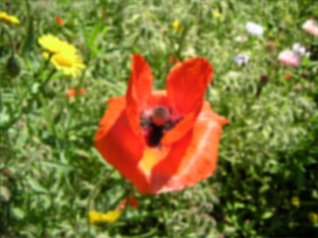The more blur you want, the bigger the filter has to be, or you can apply the same small blur filter multiple times.

If your kernel is an entire box filled with the same value (with appropriate scaling factor so all elements sum to 1.0), then the blur is called a box blur. If you want a very large box blur, then the naive convolution code in this tutorial is too slow. But you can implement it easily with a much faster algorithm: Since every value has the same factor, you can go loop through the pixels of the image line by line, and sum N values (with N the width of the box) and divide through the appropriate scaling factor. Then for every next pixel, you add the value of the next pixel that appears in the box, and subtract the value from the leftmost pixel that disappears from the box. After doing this for each scanline horizontally, do the same vertically (to optimize use of the CPU cache, when doing it vertically, ensure that you still implement it in such way that you still operate in scanline order in practice rather than per columns, so store a sum per column). This all requires care with how you treat the edges (where the box is partially out of bounds) and the case where the image is smaller than the box. No code is given here as it goes a bit beyond the scope of this tutorial.

## Gaussian Blur

In the blurring above, the kernel we used is rather harsh. A much smoother blur is achieved with a gaussian kernel. With a gaussian kernel, the value exponentially decreases as we go away from the center. The formula is: G(x) = exp(-x * x / 2 * sigma * sigma) / sqrt(2 * pi * sigma * sigma)

For 2D, you apply this formula in the X direction, then in the Y direction (it is separable), or combined it gives: G(x, y) = exp(-(x * x + y * y) / (2 * sigma * sigma)) / (2 * pi * sigma * sigma)

In the formulas:
*) sigma determines the radius (in theory the radius is in finite, but in practice due to the exponential backoff there is a point where it becomes too small to see, the larger sigma is the further away this is) *) x and y must be coordinates such that 0 is in the center of the kernel

The above formula tells hwo to make arbitrarily large kernels. However, here are simple examples that can be used immediately:

Approximation to 3x3 kernel:

#define filterWidth 3 #define filterHeight 3 double filter[filterHeight][filterWidth] = { 1, 2, 1, 2, 4, 2, 1, 2, 1, }; double factor = 1.0 / 16.0; double bias = 0.0;

Approximation to 5x5 kernel:

#define filterWidth 5 #define filterHeight 5 double filter[filterHeight][filterWidth] = { 1, 4, 6, 4, 1, 4, 16, 24, 16, 4, 6, 24, 36, 24, 6, 4, 16, 24, 16, 4, 1, 4, 6, 4, 1, }; double factor = 1.0 / 256.0; double bias = 0.0;

An exact intead of approximate example:

#define filterWidth 3 #define filterHeight 3 double filter[filterHeight][filterWidth] = { 0.077847, 0.123317, 0.077847, 0.123317, 0.195346, 0.123317, 0.077847, 0.123317, 0.077847, }; double factor = 1.0; double bias = 0.0;

For larger radius (such as you can try in a painting program that has gaussian blur), you need larger kernels. The naive convolution implementation like used in this tutorial would become too slow in practice for large radius gaussian blurs. But there are solutions to that: using the Fourier Transform as described in the Fourier Transform tutorial of this series, or an even faster approximation: The fast approximation involves doing multiple box blurs in a row, three in a row approximated it already very well. How to do a fast box blur is explained in the previous chapter. The reason this works is that a gaussian distribution arises naturally from a sum of other processes.

## Motion Blur

Motion blur is achieved by blurring in only 1 direction. Here's a 9x9 motion blur filter:

 ```#define filterWidth 9 #define filterHeight 9 double filter[filterHeight][filterWidth] = { 1, 0, 0, 0, 0, 0, 0, 0, 0, 0, 1, 0, 0, 0, 0, 0, 0, 0, 0, 0, 1, 0, 0, 0, 0, 0, 0, 0, 0, 0, 1, 0, 0, 0, 0, 0, 0, 0, 0, 0, 1, 0, 0, 0, 0, 0, 0, 0, 0, 0, 1, 0, 0, 0, 0, 0, 0, 0, 0, 0, 1, 0, 0, 0, 0, 0, 0, 0, 0, 0, 1, 0, 0, 0, 0, 0, 0, 0, 0, 0, 1, }; double factor = 1.0 / 9.0; double bias = 0.0;```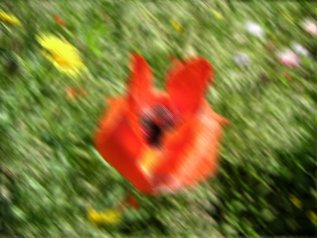It's as if the camera is moving from the top left to the bottom right, hence the name.

## Find Edges

A filter to find the horizontal edges can look like this:

 ```#define filterWidth 5 #define filterHeight 5 double filter[filterHeight][filterWidth] = { 0, 0, -1, 0, 0, 0, 0, -1, 0, 0, 0, 0, 2, 0, 0, 0, 0, 0, 0, 0, 0, 0, 0, 0, 0, }; double factor = 1.0; double bias = 0.0;```

A filter of 5x5 instead of 3x3 was chosen, because the result of a 3x3 filter is too dark on the current image. Note that the sum of all the elements is 0 now, which will result in a very dark image where only the edges it detected are colored.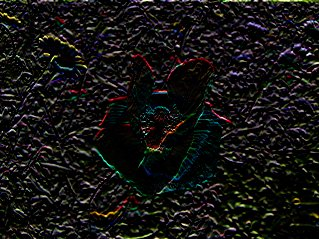The reason why this filter can find horizontal edges, is that the convolution operation with this filter can be seen as a sort of discrete version of the derivative: you take the current pixel and subtract the value of the previous one from it, so you get a value that represents the difference between those two or the slope of the function.

Here's a filter that'll find vertical edges instead, and uses both pixel values below and above the current pixel:

 ```#define filterWidth 5 #define filterHeight 5 double filter[filterHeight][filterWidth] = { 0, 0, -1, 0, 0, 0, 0, -1, 0, 0, 0, 0, 4, 0, 0, 0, 0, -1, 0, 0, 0, 0, -1, 0, 0, }; double factor = 1.0; double bias = 0.0;```Here's yet another possible filter, one that's good at finding edges of 45°. The values '-2' were chosen for no particular reason at all, just make sure the sum of the values is 0.

 ```#define filterWidth 5 #define filterHeight 5 double filter[filterHeight][filterWidth] = { -1, 0, 0, 0, 0, 0, -2, 0, 0, 0, 0, 0, 6, 0, 0, 0, 0, 0, -2, 0, 0, 0, 0, 0, -1, }; double factor = 1.0; double bias = 0.0;```And here's a simple edge detection filter that detects edges in all directions:

 ```#define filterWidth 3 #define filterHeight 3 double filter[filterHeight][filterWidth] = { -1, -1, -1, -1, 8, -1, -1, -1, -1 }; double factor = 1.0; double bias = 0.0;```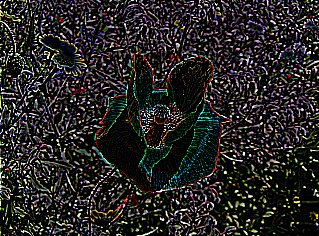## Sharpen

To sharpen the image is very similar to finding edges, add the original image, and the image after the edge detection to each other, and the result will be a new image where the edges are enhanced, making it look sharper. Adding those two images is done by taking the edge detection filter from the previous example, and incrementing the center value of it with 1. Now the sum of the filter elements is 1 and the result will be an image with the same brightness as the original, but sharper.

 ```#define filterWidth 3 #define filterHeight 3 double filter[filterHeight][filterWidth] = { -1, -1, -1, -1, 9, -1, -1, -1, -1 }; double factor = 1.0; double bias = 0.0;```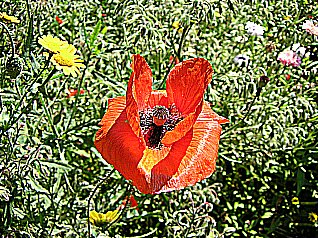Here's a more subtle sharpen filter:

 ```#define filterWidth 5 #define filterHeight 5 double filter[filterHeight][filterWidth] = { -1, -1, -1, -1, -1, -1, 2, 2, 2, -1, -1, 2, 8, 2, -1, -1, 2, 2, 2, -1, -1, -1, -1, -1, -1, }; double factor = 1.0 / 8.0; double bias = 0.0;```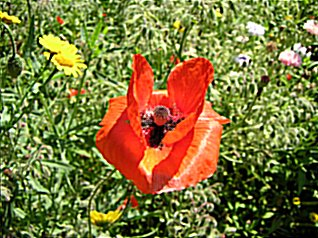Here's a filter that shows the edges excessively:

 ```#define filterWidth 3 #define filterHeight 3 double filter[filterHeight][filterWidth] = { 1, 1, 1, 1, -7, 1, 1, 1, 1 }; double factor = 1.0; double bias = 0.0;```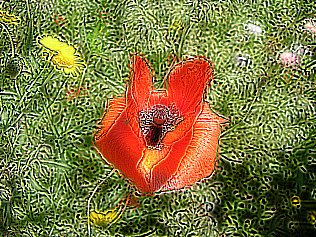## Emboss

An emboss filter gives a 3D shadow effect to the image, the result is very useful for a bumpmap of the image. It can be achieved by taking a pixel on one side of the center, and subtracting one of the other side from it. Pixels can get either a positive or a negative result. To use the negative pixels as shadow and positive ones as light, for a bumpmap, a bias of 128 is added to the image. Now, most parts of the image will be gray, and the sides will be either dark gray/black or bright gray/white.

For example here's an emboss filter with an angle of 45°:

 ```#define filterWidth 3 #define filterHeight 3 double filter[filterHeight][filterWidth] = { -1, -1, 0, -1, 0, 1, 0, 1, 1 }; double factor = 1.0; double bias = 128.0;```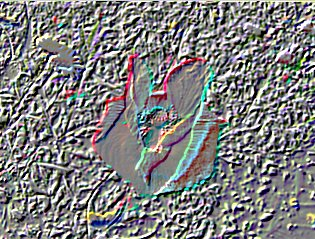If you really want to use it as bumpmap, grayscale it:Here's a much more exaggerated emboss filter:

 ```#define filterWidth 5 #define filterHeight 5 double filter[filterHeight][filterWidth] = { -1, -1, -1, -1, 0, -1, -1, -1, 0, 1, -1, -1, 0, 1, 1, -1, 0, 1, 1, 1, 0, 1, 1, 1, 1 }; double factor = 1.0; double bias = 128.0;```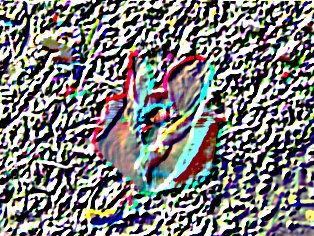## Mean and Median Filter

Both the Mean Filter and the Median Filter can be used to remove noise from an image. A Mean Filter is a filter that takes the average of the current pixel and its neighbors, for example if you use its 8 neighbors it becomes the filter with kernel:

 ```#define filterWidth 3 #define filterHeight 3 double filter[filterHeight][filterWidth] = { 1, 1, 1, 1, 1, 1, 1, 1, 1 }; double factor = 1.0 / 9.0; double bias = 0.0;```

This is an ordinary blur filter. We can test it on the following image with so called "Salt and Pepper" Noise: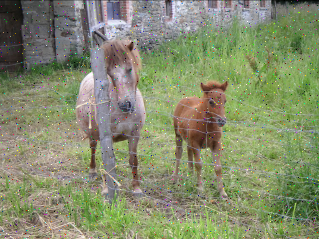When applied, it gives a blurry result:The Median Filter does somewhat the same, but, instead of taking the mean or average, it takes the median. The median is gotten by sorting all the values from low to high, and then taking the value in the center. If there are two values in the center, the average of these two is taken. A median filter gives better results to remove salt and pepper noise, because it completely eliminates the the noise. With an average filter, the color value of the noise particles are still used in the average calculations, when taking the median you only keep the color value of one or two healthy pixels. The median filter also reduces the image quality however.

Such a median filter can't be done with a convolution, and a sorting algorithm is needed, in this case combsort was chosen, which is a relatively fast sorting algorithm.

To get the median of the current pixel and its 8 neighbors, set filterWidth and filterHeight to 3, but you can also make it higher to remove larger noise particles.

The arrays red, green and blue will contain the values of the current pixel and all of its neighbors, and these are the arrays that'll be sorted by the sorting algorithm to be able to take the median value. The main function applies the filter, calculates the medians and then draws the result.

 ```#define filterWidth 3 #define filterHeight 3 //color arrays int red[filterWidth * filterHeight]; int green[filterWidth * filterHeight]; int blue[filterWidth * filterHeight]; int selectKth(int* data, int s, int e, int k); int main(int argc, char *argv[]) { //load the image into the buffer unsigned long w = 0, h = 0; std::vector image; loadImage(image, w, h, "pics/noise.png"); std::vector result(image.size()); //set up the screen screen(w, h, 0, "Median Filter"); ColorRGB color; //the color for the pixels //apply the filter for(int y = 0; y < h; y++) for(int x = 0; x < w; x++) { int n = 0; //set the color values in the arrays for(int filterY = 0; filterY < filterHeight; filterY++) for(int filterX = 0; filterX < filterWidth; filterX++) { int imageX = (x - filterWidth / 2 + filterX + w) % w; int imageY = (y - filterHeight / 2 + filterY + h) % h; red[n] = image[imageY * w + imageX].r; green[n] = image[imageY * w + imageX].g; blue[n] = image[imageY * w + imageX].b; n++; } int filterSize = filterWidth * filterHeight; result[y * w + x].r = red[selectKth(red, 0, filterSize, filterSize / 2)]; result[y * w + x].g = green[selectKth(green, 0, filterSize, filterSize / 2)]; result[y * w + x].b = blue[selectKth(blue, 0, filterSize, filterSize / 2)]; } //draw the result buffer to the screen for(int y = 0; y < h; y++) for(int x = 0; x < w; x++) { pset(x, y, result[y * w + x]); } //redraw & sleep redraw(); sleep(); }```

The array contains the value of every color in the rectangular area you're working on, but it's not sorted so you can't immediatly take the median of it. Sorting it and taking the center element is one way, but it's in theory faster to use a selection algorithm to select the k-th largest element, with k = size / 2. This is implemented below with a very simple selection algorithm. It's possible to use the standard C++ function nth_element instead, which would be simpler and faster, but we're implementing all algorithms ourselves in this tutorial. Note that, unlike the statistical definition of median this will not take the average of two elements in case of even array but just take one of them.

 ```// selects the k-th largest element from the data between start and end (end exclusive) int selectKth(int* data, int s, int e, int k) // in practice, use C++'s nth_element, this is for demonstration only { // 5 or less elements: do a small insertion sort if(e - s <= 5) { for(int i = s + 1; i < e; i++) for(int j = i; j > 0 && data[j - 1] > data[j]; j--) std::swap(data[j], data[j - 1]); return s + k; } int p = (s + e) / 2; // choose simply center element as pivot // partition around pivot into smaller and larger elements std::swap(data[p], data[e - 1]); // temporarily move pivot to the end int j = s; // new pivot location to be calculated for(int i = s; i + 1 < e; i++) if(data[i] < data[e - 1]) std::swap(data[i], data[j++]); std::swap(data[j], data[e - 1]); // recurse into the applicable partition if(k == j - s) return s + k; else if(k < j - s) return selectKth(data, s, j, k); else return selectKth(data, j + 1, e, k - j + s - 1); // subtract amount of smaller elements from k }```

Here's again the noisy image:The 3x3 median filter removes its noise:Higher sizes of filters go pretty slow, because the code is very unoptimized. More specialized, much faster algorithms for 2D median filter exists but that's beyond the scope of this tutorial. The results of higher sizes are somewhat artistic, so here is the result of different sizes:

5x5: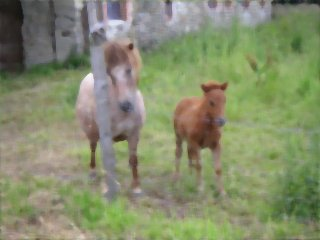9x9: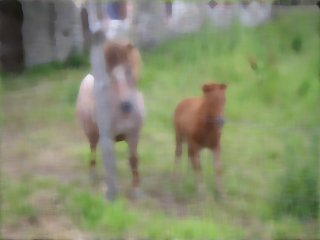15x15:Side note: The median algorithm implementation above is very slow. Whether using C++'s nth_element function or the toy "selectKth" here, both provide little benefit for a median of 9 or 25 numbers. No matter what the theoretical complexity of the algorithm on large N is, if you only operate on a certain small finite sized input, you need to take what works best for that input size.

If you want to implement, say, median with 3x3, then you get the fastest solution by using a hardcoded sorting network of size 9 of which you take the middle output to get the median. Then apply this for every output pixel to the corresponding 9 input pixels, and this all per color channel. The advantage of hardcoded is that the algorithm does not need to contain conditionals that depend on the size of the input (conditionals, like ifs and the for loop conditions, are very slow for CPUs as they interrupt the pipeline). No code is provided here since an efficiant practical implementation is beyond the scope of this tutorial. If interested, look up sorting network, it is a hardcoded series of swaps of two numbers depending on which is the maximum, find one that is proven to be optimal for the desired input size. Then since we don't want to fully sort but only take the median element, you can leave out every swap that does not contribute to the middle element output, and replace any swap of which only one output contributes to the middle output element with either min or max. That will give the theoretically fastest possible implementation, speedups on top of that can only be to make use of parallelism and/or better CPU instructions.

## Conclusion

This article contained code to apply convolution filters on images, and showed a few different filters and their result. These are only the very basics of image filtering, with bigger filters and a lot of tweaking you can get much better filters.

The Fourier Transform article shows a different way to filter images, in the frequency domain. There Low Pass, High Pass and Band Pass filters are discussed.